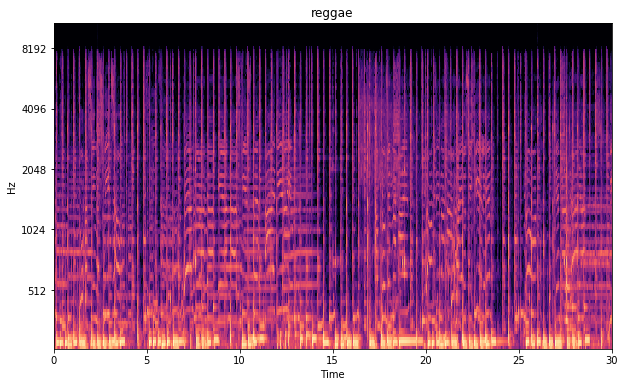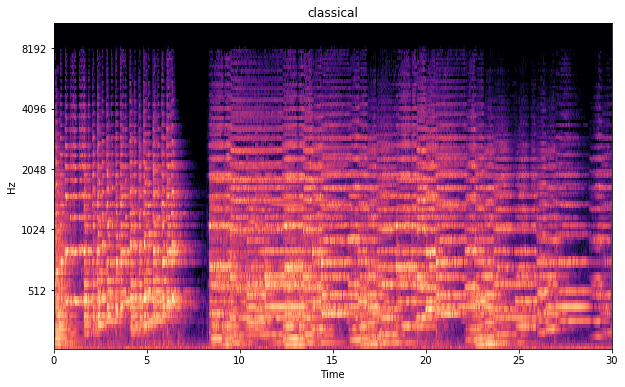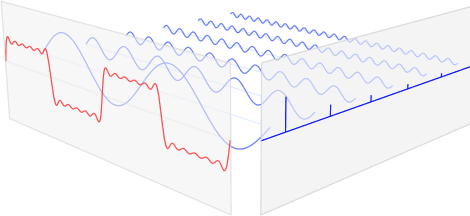Deep learning music classifier part 2. Computer says no!

June 4, 2017Recap Last post I described what my motivations were to start building a music classifier, or at least attempt to build one. The post also described how I collected a dataset, extracted important features and clustered the data based on their variance. You can read the previous post here. This post describes how I got my feet wet with classifying music. Spotify kind of sorted the data I’ve downloaded by genre.

Deep learning music classifier part 1. 30 seconds disco!

May 12, 2017Introduction As a nerd I am fascinated by the deep learning hype. Out of interest I have been following some courses, reading blogs and watched youtube video’s about the topic. Before diving into the content, I really thought this was something solely for the great internet companies and that it was not a subject us mortals could understand. While reading and learning more about it I’ve come to the insight that making use of deep learning techniques is not only something the internet giants and scientists can do.

What should be explained in the Dutch SBR-B Guideline!

May 7, 2017

The Dutch SBR guideline is intended to help you process vibration data and help you determine when a vibration signal can cause discomfort to persons. It seems to me however, that the SBR-B guideline does not have the intention to be understood. They seem to help you by making a super abstract of scientific papers and by giving you a few keywords so you can Google it yourself. This post will elaborate on two formula’s given in the guideline.

Understanding the Fourier Transform by example

April 23, 2017In the last couple of weeks I have been playing with the results of the Fourier Transform and it has quite some interesting properties that initially were not clear to me. In this post I summarize the things I found interesting and the things I’ve learned about the Fourier Transform. Application The Fourier Transformation is applied in engineering to determine the dominant frequencies in a vibration signal. When the dominant frequency of a signal corresponds with the natural frequency of a structure, the occurring vibrations can get amplified due to resonance.

Writing a fourth order Runga Kutta solver for a vibrations problem in Python (Part 1)

April 13, 2017

Problem If you want to solve a vibrations problem with a force acting on the system you often need to find the solution in nummerical algorithms. Say you have got a single degree of freedom mass spring system as shown in the figure below. SDOF damped mass spring system The differential equation of this system is: $mu'' + cu' + ku = F$ When the force that acts on the system is a function, this problem can be solved with symbolical maths by solving the differential equation.

Writing a fourth order Runga Kutta solver for a vibrations problem in Python (Part 2)

April 13, 2017

This post continues where part 1 ended. In order to increase the accuracy of our function solver we are going to use a 4th order Runga Kutta algorithm. The basics are the same as with the Euler method. However the dy part of the 4th order method is more accurately computed. Definition The incremental values of this method are defined as: $y_{n+1} = y_{n} + \frac{h}{6}(k_{1} + 2k_{2} +2k_{3} + k_{4})$ $t_{n+1} = t_{n} + h$ With the factors k1 - k4 being:

Writing a fourth order Runga Kutta solver for a vibrations problem in Python (Part 3)

April 13, 2017

This post continues where part 2 ended. The Runga Kutta algorithm described in last post is only able to solve first order differential equations. The differential equation (de) for a single mass spring vibrations problem is a second order de. $mu'' + cu' + ku = F$ Note that in this equation: u” = acceleration a u’ = velocity v u = displacement Before we can solve it with a Runga Kutta algorithm we must rewrite the base equation to a system of two first order ode’s.

Python 1D FEM Example 3

March 12, 2017

Python 1D FEM Example 3. Simple code example for anaStruct. # if using ipython notebook %matplotlib inline from anastruct.fem.system import SystemElements # Create a new system object. ss = SystemElements(EA=15000, EI=5000) # Add beams to the system. ss.add_element(location=[[0, 0], [0, 5]]) ss.add_element(location=[[0, 5], [5, 5]]) ss.add_element(location=[[5, 5], [5, 0]]) # Add a fixed support at node 1. ss.add_support_fixed(node_id=1) # Add a rotational spring at node 4. ss.add_support_spring(node_id=4, translation=3, k=4000) # Add loads.

Python 1D FEM Example 2

February 12, 2017

Example 2: Truss framework Simple code example for anaStruct. # if using ipython notebook %matplotlib inline import math from anastruct.fem.system import SystemElements # Create a new system object. ss = SystemElements(EA=5000) # Add beams to the system. ss.add_truss_element(location=[[0, 0], [0, 5]]) ss.add_truss_element(location=[[0, 5], [5, 5]]) ss.add_truss_element(location=[[5, 5], [5, 0]]) ss.add_truss_element(location=[[0, 0], [5, 5]], EA=5000 * math.sqrt(2)) # get a visual of the element ID's and the node ID's ss.show_structure() # add hinged supports at node ID 1 and node ID 2 ss.

Python 1D FEM Example 1

January 12, 2017

Example 1: Framework Simple code example for anaStruct. # if using ipython notebook %matplotlib inline from anastruct.fem.system import SystemElements # Create a new system object. ss = SystemElements() # Add beams to the system. ss.add_element(location=[[0, 0], [3, 4]], EA=5e9, EI=8000) ss.add_element(location=[[3, 4], [8, 4]], EA=5e9, EI=4000) # get a visual of the element IDs and the node IDs ss.show_structure() # add loads to the element ID 2 ss.q_load(element_id=2, q=-10) # add hinged support to node ID 1 ss.

(c) 2019 Ritchie Vink.